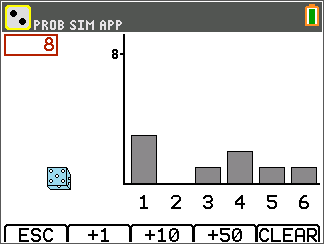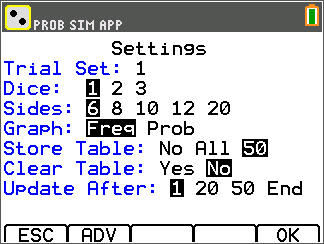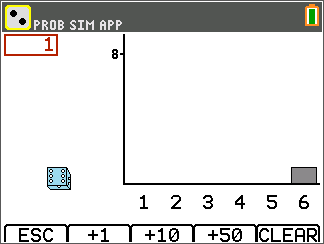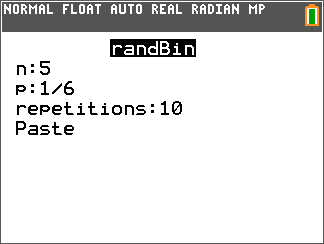••• ##### Device
• TI-84 Plus
• TI-84 Plus Silver Edition
•TI-84 Plus C Silver Edition
•TI-84 Plus CE
• ##### Report an Issue

Algebra II: Binomial Probabilities
by Texas Instruments#### Overview

Students simulate rolling a die and keeping track of the numbers of successes and failures by using the randBin command.

#### Key Steps

•In Problem 1, students can either use the Roll Dice simulation of the Prob Sim app or roll a real die to investigate binomial probabilities.

Each student rolls the die five times and records the result where a 6 is a success. They are to repeat this experiment nine more times. They track their results in a table provided on their worksheets.

•In a second table, they record the number and percentage of successes in each of the 10 experiments. The number of successes should sum to 10 and the percent of successes (in decimal form) should sum up to 1, this will represent the experimental binomial probabilities.

•Students will use the randBin command that simulates binomial experiments. This will help students see how the simulated results compare to their actual results.